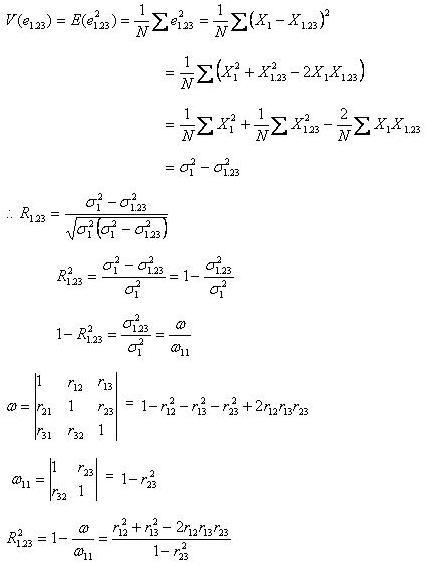# Statistics Assignment Help With Coefficient Of Multiple Correlation

## 8.14. Coefficient Of Multiple Correlation:

In a tri-variate distribution in which each of the variable X1,X2, and X3 has N observations, the multiple correlation coefficient of X1 on X2 and X3 usually denoted by R 1.23 is the simple correlation coefficient between X1 and the joint effect of X2 and X3 on X1. In other words R1.23 is the correlation coefficient between X1 and its estimated value as given by the plane of regression of X1 on X2 and X3.Also

###This formula expresses the multiple correlation coefficient in terms of the total correlation coefficients between the pairs of variables.